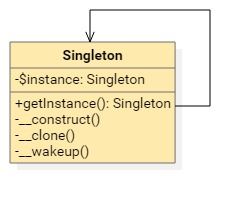• 我们中的有些人，注定要在日常生活的点滴中去寻找生命的意义。---- 《生活大爆炸》|

# 单例模式

5年前 (2017-06-09) 376次浏览

## 1 问题

```class Database
{
public \$db = null;
public function __construct(\$config = array())
{
\$dsn = sprintf('mysql:host=%s;dbname=%s', \$config['db_host'], \$config['db_name']);
\$this->db = new PDO(\$dsn, \$config['db_user'], \$config['db_pass']);
}
}```

```\$config = array(
'db_name' => 'test',
'db_host' => 'localhost',
'db_user' => 'root',
'db_pass' => 'root'
);

\$db1 = new Database(\$config);
var_dump(\$db1);
\$db2 = new Database(\$config);
var_dump(\$db2);
\$db3 = new Database(\$config);
var_dump(\$db3);```

```object(Database)
public 'db' => object(PDO)
object(Database)
public 'db' => object(PDO)
object(Database)
public 'db' => object(PDO)```

## 2 解决

```class Database
{
// 声明\$instance 为私有静态类型，用于保存当前类实例化后的对象
private static \$instance = null;
// 数据库连接句柄
private \$db = null;

// 构造方法声明为私有方法，禁止外部程序使用 new 实例化，只能在内部 new
private function __construct(\$config = array())
{
\$dsn = sprintf('mysql:host=%s;dbname=%s', \$config['db_host'], \$config['db_name']);
\$this->db = new PDO(\$dsn, \$config['db_user'], \$config['db_pass']);
}

// 这是获取当前类对象的唯一方式
public static function getInstance(\$config = array())
{
// 检查对象是否已经存在，不存在则实例化后保存到\$instance 属性
if(self::\$instance == null) {
self::\$instance = new self(\$config);
}
return self::\$instance;
}

// 获取数据库句柄方法
public function db()
{
return \$this->db;
}

// 声明成私有方法，禁止克隆对象
private function __clone(){}
// 声明成私有方法，禁止重建对象
private function __wakeup(){}
}```

```\$config = array(
'db_name' => 'test',
'db_host' => 'localhost',
'db_user' => 'root',
'db_pass' => 'root'
);

\$db1 = Database::getInstance(\$config);
var_dump(\$db1);
\$db2 = Database::getInstance(\$config);
var_dump(\$db2);
\$db3 = Database::getInstance(\$config);
var_dump(\$db3);```

```object(Database)
private 'db' => object(PDO)
object(Database)
private 'db' => object(PDO)
object(Database)
private 'db' => object(PDO)```

`\$db = database::getInstance(\$config)->db();`

## 3 特点

```class Singleton
{
private static \$instance = null;

public static function getInstance()
{
if(self::\$instance == null) {
self::\$instance = new self();
}
return self::\$instance;
}

private function __construct(){}
private function __clone(){}
private function __wakeup(){}
}```

`\$instance`用以保存类的实例化，`getInstance()`方法提供给外部本类的实例化对象：